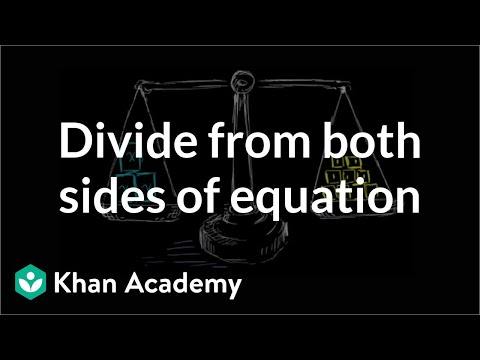Video

# Modeling with one-step equations (Full video)

Description: Learn how to write basic equations to model real-world situations. For \$42.50 total, they can buy p boxes of pizza. So 42.50 that's the total amount they spent on pizza and if I wanted to figure out how many boxes of pizza they could buy, I could divide the total amount they spend, I could divide that by the price per box. So 42.50, but what's another way of thinking about the total they spend?

### Other videos you might be interested in### Using Substitution to Understand Equations and Inequalities (Full video)

#### Student Achievement Partners